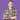# Introducing Big O Notation

March 26, 2019

As programmers, we write code to tell computers what to do. The computer translates this code into operations, and then executes those operations with some amount of space in memory in some amount of time. We say that one block of code is more efficient than another if it uses less space or time.

Efficient: performing or functioning in the best possible manner with the least waste of time and effort (dictionary.com)

Suppose we want to to find the minimum value from an array of values.

``````function getMinimum(values) {
let minimum = Infinity

values.forEach(value => {
if (value < minimum) {
minimum = value
}
})

return minimum
}``````

With this function, we step through the array of values; update the minimum whenever we find a new minimum; and, then return the minimum.

``````function getMinimum(values) {
// Sort values from lowest to highest
values.sort((a, b) => a - b)
return values || Infinity
}``````

With this function, we sort the list so the minimum is the first element, and then return the first element.

But, sorting is expensive, since we may need to compare every value against every other value. Therefore, it is more efficient to use the first function, even if there are more lines of code.

With software, efficiency means using less space or time.

## Measuring Efficiency

To measure efficiency, we can use Big O Notation. This notation measures the worst-case complexity of space or time for a function in relation to its inputs.

Let’s look at some examples.

### O(1)

With `O(1)` time, the work does not increase in relation to the input.

``````function logName(name) {
console.log(name)
}``````

In this case, we will always call `console.log()` one time. It doesn’t matter what `name` is.

``````function logNameThreeTimes(name) {
console.log(name)
console.log(name)
console.log(name)
}``````

In this case, we will always call `console.log()` three times. Again, `name` does not matter.

We say that these functions run in constant time.

### O(n)

With `O(n)` time, the work increases linearly with the input.

``````function logUserNames(users) {
users.forEach(user => console.log(user.name))
}``````

In this case, we call `console.log()` once per user.

``````function logUserNamesThreeTimes(users) {
users.forEach(user => console.log(user.name))
users.forEach(user => console.log(user.name))
users.forEach(user => console.log(user.name))
}``````

In this case, we call `console.log()` three times per user, so the work still grows linearly.

But, what happens when we add more logic to our code?

``````function logPublicUserNames(users) {
users.forEach(user => {
if (user.isPublic) {
console.log(user.name)
}
})
}``````

In this case, we may need to log 0 names, 1 name, some names, or every name. So, we assume the worst-case scenario: log every name. Thus, we still have `O(n)` time.

We say these functions run in linear time.

### O(n^2)

With `O(n^2)` time, the work increases quadratically with the inputs.

``````function countAppearances(values) {
const appearancesByValue = {}

values.forEach(value => {
appearancesByValues[value] = 0
values.forEach(comparison => {
if (value === comparison) {
appearancesByValues[value]++
}
})
})

return appearancesByValue
}``````

In this case, we step through all values for each value we check. If we increase the length of values by 1, we have to check every value yet again.

We say this function runs in quadratic time.

As you may have guessed, this function can be made more efficient:

``````function countAppearances(values) {
const appearancesByValue = {}

values.forEach(value => {
// hasOwnProperty() runs in O(1) time
if (appearancesByValue.hasOwnProperty(value)) {
appearancesByValues[value]++
} else {
appearancesByValues[value] = 1
}
})

return appearancesByValue
}``````

By stepping through all values only once, we have reduced this function to `O(n)` time. Much better!

### O(n^c)

In general, the more loops you nest, the worse your efficiency.

``````// O(n^3)
values.forEach(value1 => {
values.forEach(value2 => {
values.forEach(value3 => {
// Do some work
})
})
}

// O(n^4)
values.forEach(value1 => {
values.forEach(value2 => {
values.forEach(value3 => {
values.forEach(value4 => {
// Do some work
})
})
})
}

// and so on ...``````

We say these functions run in polynomial time.

### And so on…

Constant, linear, quadratic, and polynomial times are just the beginning. As you dig deeper into complexity, you will see many more - like `O(logn)` for Binary search.

## Don’t Forget Space

As mentioned earlier, Big O Notation can also be applied to space. That is, the memory required to execute the code, excluding the input and output.

Consider a function that runs in `O(1)` time of 10 seconds, but requires up to 10 gigabytes of memory to execute. Is it worth sacrificing some time to reduce the space?

## The Worst Factor

With Big O Notation, efficiency is measured by the worst factor. If part of a function takes `O(n)` time, but another takes `O(n^2)` time, then the whole function takes `O(n^2)` time.

Therefore, to improve efficiency, we must reduce the worst factor.

## Writing More Efficient Code

Once we have a solution to a problem, we can make our code more efficient by reducing the worst factor. After all, there can only be minor improvements if we only optimize the good parts.

Some quick tips:

• Avoid loops within loops
• Use objects for `O(1)` lookup
• Check the complexity of functions you use
• Catch easy cases as soon as possible

By finding and reducing the worst factor, we can write more efficient code.